# Sequence

of elements of a given set

A function defined on the set of positive integers whose range is contained in the set considered.

An element, or term, of a sequence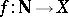, whereis the set of positive integers andis the given set, is an ordered pair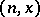,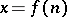,,, denoted by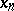. The positive integeris called the number (or index) of the termand the elementis called its value. The sequenceis usually denoted by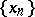or,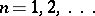.

The set of elements of a sequence is always countable; moreover, two different terms of a sequence are different at least with respect to their indices. The set of values of the elements of a sequence may be finite; e.g., the set of values of any stationary sequence, i.e. of a sequenceall elements of which have one and the same value,consists of one element.

If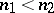, then the term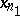of a sequenceis called a predecessor of an element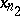, and the termis called a successor of. Thus, the set of elements of a sequence is ordered.

Various types of sequences are encountered in many branches of mathematics. They help to describe many properties of objects under study. For instance, ifis a topological space, then among the sequences of points of it an important role is played by convergent sequences, i.e. by sequences that have a limit in this space. Convergent sequences are convenient (at least when a countable base is available) for the description of such properties as compactness, existence of a limit of a mapping, continuity of a mapping, etc. If all elements of a sequence of some objects (points, sets, mappings, etc.) have a certain property, it is often important to find out whether this property is preserved at a limit point of this sequence. For example, to consider the behaviour of such properties as measurability, continuity, differentiability, and integrability under limit transition for different types of convergence of functions (pointwise convergence, convergence almost-everywhere, uniform convergence, convergence in measure, convergence in the mean, etc.).

Sometimes a mapping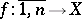from a finite set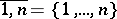of positive integers into a setis called a finite sequence and is denoted by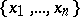, where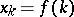,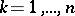. A sequence can be given by a formula for its general term (e.g. an arithmetical sequence), by a recurrence formula (e.g. the sequence of Bernoulli numbers) or simply by a verbal description with a certain degree of efficiency (e.g. the sequence of all positive prime integers in ascending order). See also Double sequence; Multiple sequence. The generalization of the notion of a sequence is that of a generalized sequence.

How to Cite This Entry:
Sequence. L.D. Kudryavtsev (originator), Encyclopedia of Mathematics. URL: http://www.encyclopediaofmath.org/index.php?title=Sequence&oldid=17138
This text originally appeared in Encyclopedia of Mathematics - ISBN 1402006098